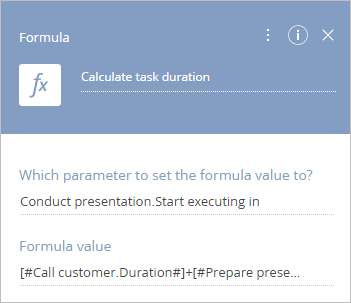# [Formula] process element

Products
All Creatio products

With the Formula process element, you can use the calculation results as parameter values for other elements.

For example, you can determine the start time of the next task based on the duration of the preceding tasks.

The Formula element is also used to define conditions for moving down the conditional flows.

Specify the parameter values in the Formula setup area (Fig. 1).

Fig. 1 The Formula element setup areaYou can populate these fields using the process parameters.

Enter the element caption at the top of the element setup area. The caption is displayed on the process diagram.

Which parameter to set the formula value to? – Specify the parameter whose value will contain the calculation result. For example, specify the Duration parameter of the Perform task process element if the formula is used to calculate the duration of a task. You can select a parameter whose value is a number, date and/or time, as well as text and boolean parameters.

Attention. The resulting data type will correspond to the data type of the parameter specified in the Set parameter value field. When adding the Formula process element, you can only specify the parameters that already exist in the process. Each Formula process element performs calculations for one parameter only.

Formula value – use the parameter value window to construct the formula. The formula value is entered in the text field. The formula can contain elements from the Process elements, Process parameters, System settings, Lookup, System variables, Functions, and Date and time tabs.